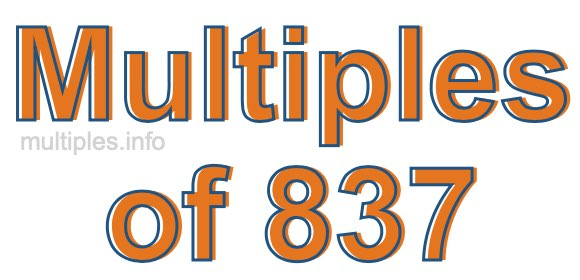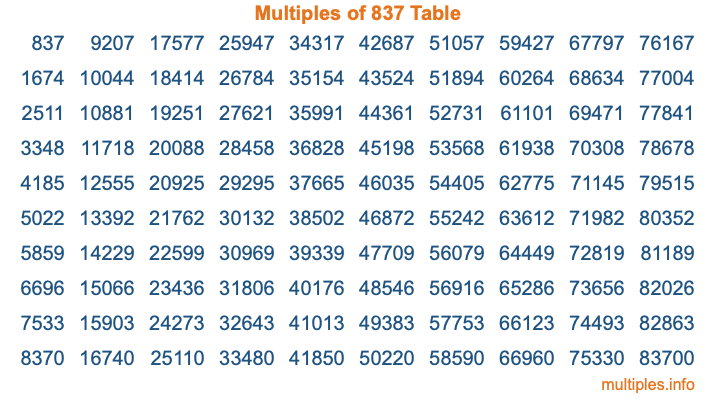Multiples of 837Welcome to the Multiples of 837 page. Here we will first teach you everything you will ever need to know about the multiples of 837, and then give you a study guide summary of everything we taught you to make sure you remember it all. Use this page to look up facts and learn information about the multiples of 837. This page will make you a multiples of eight hundred thirty-seven expert!

Definition of Multiples of 837
Multiples of 837 are all the numbers that when divided by 837 equal an integer. Each of the multiples of 837 are called a multiple. A multiple of 837 is created by multiplying 837 by an integer.

Therefore, to create a list of multiples of 837, you start with 1 multiplied by 837, then 2 multiplied by 837, then 3 multiplied by 837, and so on for as long as you want. Thus, the list of the first five multiples of 837 is 837, 1674, 2511, 3348, and 4185. To see a larger list of multiples of 837, see the printable image of Multiples of 837 further down on this page. We also have a category where you can choose any nth multiple of 837.

Multiples of 837 Checker
The Multiples of 837 Checker below checks to see if any number of your choice is a multiple of 837. In other words, it checks to see if there is any number (integer) that when multiplied by 837 will equal your number. To do that, we divide your number by 837. If the the quotient is an integer, then your number is a multiple of 837.

Is  a multiple of 837?

Least Common Multiple of 837 and ...
A Least Common Multiple (LCM) is the lowest multiple that two or more numbers have in common. This is also called the smallest common multiple or lowest common multiple and is useful to know when you are adding our subtracting fractions. Enter one or more numbers below (837 is already entered) to find the LCM.

Check out our LCM Calculator if you need more details about the Least Common Multiple or if you need the LCM for different numbers for adding and subtraction fractions.

nth Multiple of 837
As we stated above, 837 is the first multiple of 837, 1674 is the second multiple of 837, 2511 is the third multiple of 837, and so on. Enter a number below to find the nth multiple of 837.

th multiple of 837

Multiples of 837 vs Factors of 837
837 is a multiple of 837 and a factor of 837, but that is where the similarities end. All postive multiples of 837 are 837 or greater than 837. All positive factors of 837 are 837 or less than 837.

Below is the beginning list of multiples of 837 and the factors of 837 so you can compare:

Multiples of 837: 837, 1674, 2511, 3348, 4185, etc.

Factors of 837: 1, 3, 9, 27, 31, 93, 279, 837

As you can see, the multiples of 837 are all the numbers that you can divide by 837 to get a whole number. The factors of 837, on the other hand, are all the whole numbers that you can multiply by another whole number to get 837.

It's also interesting to note that if a number (x) is a factor of 837, then 837 will also be a multiple of that number (x).

Multiples of 837 vs Divisors of 837
The divisors of 837 are all the integers that 837 can be divided by evenly. Below is a list of the divisors of 837.

Divisors of 837: 1, 3, 9, 27, 31, 93, 279, 837

The interesting thing to note here is that if you take any multiple of 837 and divide it by a divisor of 837, you will see that the quotient is an integer.

Multiples of 837 Table
Below is an image of the first 100 multiples of 837 in a table. The table is in chronological order, column by column. The first column has the first ten multiples of 837, the second column has the next ten multiples of 837, and so on.The Multiples of 837 Table is also referred to as the 837 Times Table or Times Table of 837. You are welcome to print out our table for your studies.

Negative Multiples of 837
Although not often discussed or needed in math, it is worth mentioning that you can make a list of negative multiples of 837 by multiplying 837 by -1, then by -2, then by -3, and so on, to get the following list of negative multiples of 837:

-837, -1674, -2511, -3348, -4185, etc.

Multiples of 837 Summary
Below is a summary of important Multiples of 837 facts that we have discussed on this page. To retain the knowledge on this page, we recommend that you read through the summary and explain to yourself or a study partner why they hold true.

There are an infinite number of multiples of 837.

A multiple of 837 divided by 837 will equal a whole number.

837 divided by a factor of 837 equals a divisor of 837.

The nth multiple of 837 is n times 837.

The largest factor of 837 is equal to the first positive multiple of 837.

837 is a multiple of every factor of 837.

837 is a multiple of 837.

A multiple of 837 divided by a divisor of 837 equals an integer.

837 divided by a divisor of 837 equals a factor of 837.

Any integer times 837 will equal a multiple of 837.

Multiples of a Number
Here you can get the multiples of another number, all with the same attention to detail as we did for multiples of 837 on this page.

Multiples of
Multiples of 838
Did you find our page about multiples of eight hundred thirty-seven educational? Do you want more knowledge? Check out the multiples of the next number on our list!